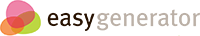# Parallel and Perpendicular Lines

## Comparing slopes

### Slope Formula The slope, m, of a line passing through two arbitrary points (x1, y1) and (x2, y2) is calculated as follows…  CHANGE IN Y'S OVER CHANGE IN X'S

The slope, m, of a line passing through two arbitrary points (x1, y1) and (x2, y2) is calculated as follows… OVER

• -5
• 1/5
• -1/5

## Practice

### y = 3x + 5;     y = -1/3 x + 5

• parallel
• perpendicular
• neither

### y = 3x + 7; y = 7x + 3

• parallel
• perpendicular
• neither

### y = 2/3 x + 8; y = -3/2 x + 8

• parallel
• perpendicular
• neither

### Which slope would make these lines perpendicular?

• -4/3
•## Slopes in equations

### Which slope matches the equation?

• y = 3x + 5
y = 3x + 9; parallel
• y = 3x + 5
y = -1/3 x +7; perpendicular
• y = 3x + 5
y = -3x -5; neither